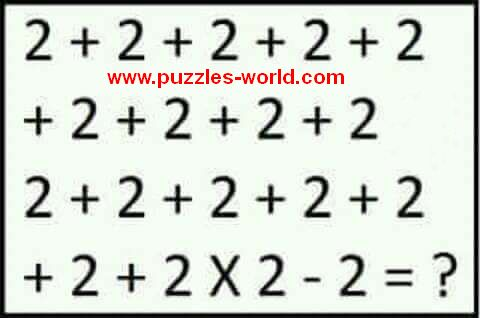# 2 + 2 + 2 + 2 + 2 + 2 + 2 + 2 + 2 2 + 2 + 2 + 2 + 2 + 2 + 2 x 2 - 2 = ?2 + 2 + 2 + 2 + 2
+ 2 + 2 + 2 + 2
2 + 2 + 2 + 2 + 2
+ 2 + 2 x 2 - 2 = ?

What will come in place of Question mark ?